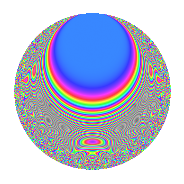# Properties

 Label 751.2.aLevel 751 Weight 2 Character orbit a Rep. character $$\chi_{751}(1,\cdot)$$ Character field $$\Q$$ Dimension 62 Newforms 2 Sturm bound 125 Trace bound 1

# Related objects

## Defining parameters

 Level: $$N$$ = $$751$$ Weight: $$k$$ = $$2$$ Character orbit: $$[\chi]$$ = 751.a (trivial) Character field: $$\Q$$ Newforms: $$2$$ Sturm bound: $$125$$ Trace bound: $$1$$ Distinguishing $$T_p$$: $$2$$

## Dimensions

The following table gives the dimensions of various subspaces of $$M_{2}(\Gamma_0(751))$$.

Total New Old
Modular forms 63 63 0
Cusp forms 62 62 0
Eisenstein series 1 1 0

The following table gives the dimensions of the cuspidal new subspaces with specified eigenvalues for the Atkin-Lehner operators.

$$751$$Dim.
$$+$$$$24$$
$$-$$$$38$$

## Trace form

 $$62q - q^{2} - 2q^{3} + 57q^{4} + 2q^{5} - 6q^{7} - 3q^{8} + 62q^{9} + O(q^{10})$$ $$62q - q^{2} - 2q^{3} + 57q^{4} + 2q^{5} - 6q^{7} - 3q^{8} + 62q^{9} - 10q^{10} - 2q^{11} - 6q^{12} + 2q^{13} - 2q^{14} - 14q^{15} + 35q^{16} + 10q^{17} + q^{18} - 2q^{19} - 4q^{20} - 6q^{21} - 6q^{22} - 8q^{23} + 22q^{24} + 60q^{25} - 4q^{26} + 4q^{27} - 36q^{28} + 4q^{29} + 2q^{30} - 22q^{31} + 3q^{32} - 8q^{34} + 4q^{35} + 47q^{36} + 6q^{37} - 6q^{38} - 16q^{39} - 52q^{40} + 18q^{41} + 4q^{42} - 6q^{43} + 12q^{44} - 4q^{45} - 32q^{46} + 20q^{47} - 10q^{48} + 64q^{49} + 15q^{50} + 14q^{51} + 12q^{52} + 12q^{53} + 60q^{54} - 6q^{55} - 18q^{56} - 2q^{57} - 28q^{58} + 8q^{59} - 40q^{60} + 8q^{61} + 24q^{62} - 20q^{63} + 9q^{64} + 6q^{65} - 36q^{66} - 8q^{67} + 42q^{68} - 34q^{69} - 32q^{70} - 26q^{71} - 17q^{72} - 12q^{73} + 72q^{74} + 34q^{75} - 24q^{76} - 6q^{77} + 18q^{78} - 62q^{79} + 42q^{80} + 78q^{81} - 12q^{83} + 2q^{84} + 26q^{85} - 16q^{86} + 38q^{87} - 50q^{88} + 42q^{89} - 32q^{90} - 14q^{91} - 32q^{92} - 18q^{93} + 10q^{94} - 4q^{95} - 12q^{96} + 14q^{97} - 5q^{98} - 10q^{99} + O(q^{100})$$

## Decomposition of $$S_{2}^{\mathrm{new}}(\Gamma_0(751))$$ into irreducible Hecke orbits

Label Dim. $$A$$ Field CM Traces A-L signs $q$-expansion
$$a_2$$ $$a_3$$ $$a_5$$ $$a_7$$ 751
751.2.a.a $$24$$ $$5.997$$ None $$-5$$ $$-8$$ $$-16$$ $$-7$$ $$+$$
751.2.a.b $$38$$ $$5.997$$ None $$4$$ $$6$$ $$18$$ $$1$$ $$-$$